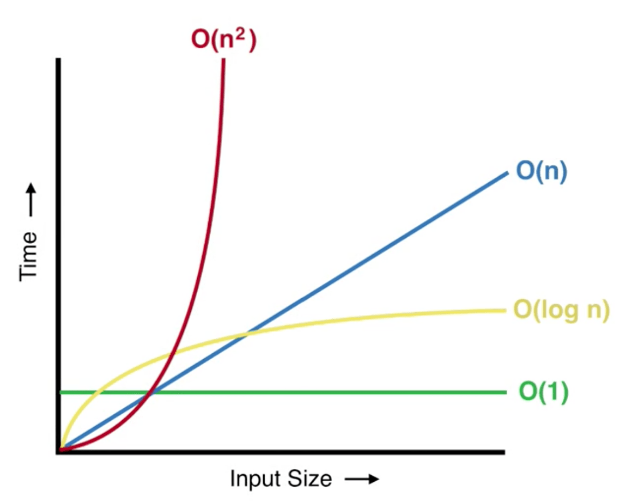# Part-6: Quadratic time complexity O(n2)

In our last tutorial we learnt about Logarithmic Time Complexity O(log n). If you have not read that article I would suggest you to read it first.

## What is Quadratic time complexity O(n2) ?

If time execution of an Algorithm is directly proportional to the squared size of the input size (n) then it is said to have Quadratic time complexity. Let's look at the diagram for such complexity:# Examples of Quadratic time complexity:

I assume that so far you read all my previous tutorials on Big O notation therefore you have basic understanding of calculating time/space complexity of an algorithm.

Let's look at the following code:

```// following loop starts from 0 index and loops until n
for(let i=0; i<=n; i++) {

// following loop starts at 0 index and runs until n
for (let j=0; j<=n; i++) {

// following statement will be repeated n * n times
console.log(i, j);
}
}

// time complexity of above loops
T(n) = O(n^2)```

let's understand above code:

• First loop repeats for n times
• According to our theory any statement that falls under this loop will also repeat for n times
• Second statement also repeat for n times therefore total time to run second loop will be n*n times
• Now, we are printing values of i, j this statement is also under the second loop. Second loop is repeated for n*n times therefore this console.log statement will also be repeated for n*n times.
• Therefore you can say that above code has O(n*n) = Quadratic Time complexity.

# Find the time complexity of the following program?

Let's analize a real code and find out the time complexity of the code below:

```function checkForDuplicate(arr) {

// following loop will repeat until n elements
for(let i=0; i<arr.length; i++) {

// statement runs for O(1) times n repetition
const tempArr = arr.slice(i+1); // O(1*n)

// following function indexOf will repeat n times for tempArr
// it will also loop for n times of above for loop therefore
// if statement will run O(n*n) times in total
if (tempArr.indexOf(arr[i]) !== -1) { // O(n*n)

// following statement runs O(1) constant time
// however it will be repeated n time due to for loop
return true; // O(1*n)
}
}

// this statement is outside for for loop
// therefore will be repeated for 1 time only
return false; // O(1)
}

checkForDuplicate([1, 5, 2, 3, 4, 5]);

// Calculating Time Complexity of above code
T(n) = O(1*n) + O(n*n) + O(1*n) + O(1)
= O(n*n)
= O(n^2) // ignoring all constants and lower terms```

In order to find Big(O) complexity of any code you have to consider all the statement that runs inside the function. You have to analysis all the statement and then figure out the bigger term for the equation.

In next tutorial, we will learn about Exponential Time Complexity O(2n).